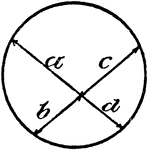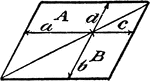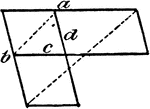### Model Of Geometric Proportions In A Circle

An illustration showing a model of a circle with intersecting chords that illustrates the following…### Model Of Geometric Proportions

An illustration showing a quadrilateral model that illustrates the following relationships: a:b = c:d,…### Model Of Geometric Proportions

An illustration showing a model that illustrates the following relationships: a:b = c:d, ad = bc. Product…